# Non Verbal Reasoning - Figure Matrix

Exercise : Figure Matrix - Section 1
Directions to Solve

In each of the following questions, find out which of the answer figures (1), (2), (3) and (4) completes the figure matrix ?

1.

Select a suitable figure from the four alternatives that would complete the figure matrix.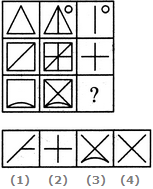1
2
3
4
Explanation:
The third figure in each row comprises of parts which are not common to the first two figures.

2.

Select a suitable figure from the four alternatives that would complete the figure matrix.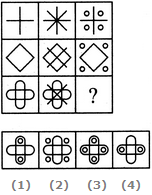1
2
3
4
Explanation:
In each row, the second figure is obtained from the first figure by adding two mutually perpendicular line segments at the centre and the third figure is obtained from the first figure by adding four circles outside the main figure.

3.

Select a suitable figure from the four alternatives that would complete the figure matrix.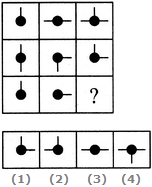1
2
3
4
Explanation:
In each row, the third figure comprises of a black circle and only those line segments which are not common to the first and the second figures.

4.

Select a suitable figure from the four alternatives that would complete the figure matrix.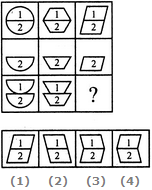1
2
3
4
Explanation:
In each column, the second figure (middle figure) is obtained by removing the upper part of the first figure (uppermost figure) and the third figure (lowermost figure) is obtained by vertically inverting the upper part of the first figure.

5.

Select a suitable figure from the four alternatives that would complete the figure matrix.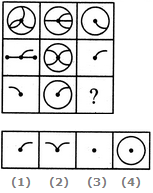1
2
3
4